﻿ Graphing linear equations - MathBootCamps

# Graphing linear equations

There are three ways you can graph linear equations: (1) you can find two points, (2) you can use the y-intercept and the slope, or (3) you can use the x- and y-intercepts. In the following guide, we will look at all three. Graphing linear equations doesn’t have to be difficult, and can even be fun once you have these methods down!

## Method 1: using two points to graph a linear equation

The graph of any linear equation like $$y = 3x + 2$$ or $$y = -x + 9$$ is a line, and only two points are needed to define a line. The idea with this method is to find two points on the line by picking values of $$x$$.

### Example

Graph the linear equation:
$$y = \dfrac{1}{3}x – 2$$

### Solution

To find two points on the line, pick any two values of $$x$$ that would be easy to work with and then find the corresponding value of $$y$$. Two easy values here would be 0 and 3 (Since the 3 will cancel with the 3 in the fraction.)

Let $$x = 0$$:

\begin{align}y &= \dfrac{1}{3}x – 2 \\ &= \dfrac{1}{3}(0) – 2\\ &= -2\end{align}

So, one point on the line is $$(x,y) = (0, –2)$$.

Let $$x = 3$$:

\begin{align}y &= \dfrac{1}{3}x – 2\\ &= \dfrac{1}{3}(3) – 2\\ &= 1 – 2\\ &= -1\end{align}

So, another point on the line is $$(x,y) = (3,–1)$$.

Once you have your two points, you can plot them and then sketch the line. It is best to use a ruler or something similar to make sure that you draw the best representation of the graph as possible.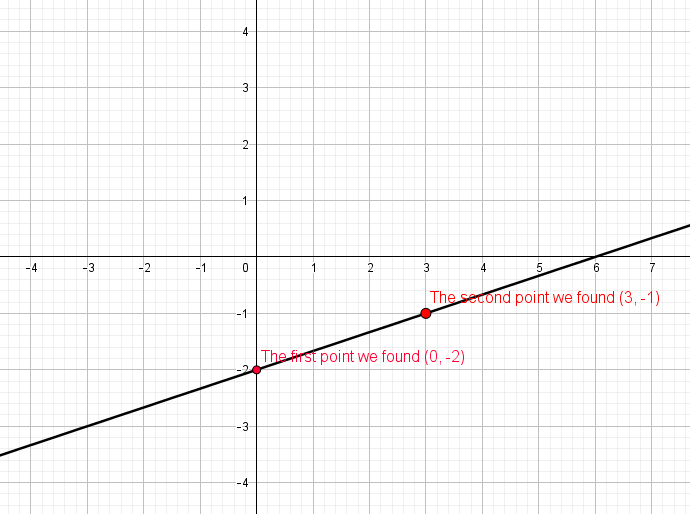TIP: You might find it useful to find three or four points on the graph so that you can sketch it more accurately. To do this, just pick more x-values to find points!

## Method 2: Use the slope and y-intercept

A linear equation written in the form $$y = mx + b$$ is said to be in slope-intercept form. This form shows the slope $$m$$ and the y-intercept $$b$$ of the graph. Knowing these two values will let you quickly draw the graph of the linear equation, as you can see in the example below.

### Example

Graph the linear equation:
$$y = \dfrac{2}{3}x + 4$$

### Solution

Since this equation is in the form $$y = mx + b$$, you know that:

• The slope is:
$$m=\dfrac{2}{3}$$
• The y-intercept is:
$$b = 4$$

Let’s look at the y-intercept first.

The y-intercept is the point where the graph crosses the y-axis (the vertical axis). So, you can plot this point as:Now consider the slope. Slope can be viewed as a rate of change: it represents the change in $$y$$ over the change in $$x$$. Sometimes, this is called “rise over run”.

$$\text{slope} = \dfrac{\text{rise}}{\text{run}} = \dfrac{\text{change in }y}{\text{change in }x}$$

For this example:

$$\text{slope} = \dfrac{2}{3} = \dfrac{\text{change in }y}{\text{change in }x}$$

This can be translated to:

$$\dfrac{2}{3} = \dfrac{\text{up 2 units}}{\text{for every 3 units right}}$$

Therefore, to find another point on the line, start with the y-intercept and go 3 unit right and 2 units up. Do this again, and you will find another point. In fact, you can keep doing this to find as many points as you think you will need to sketch a good graph.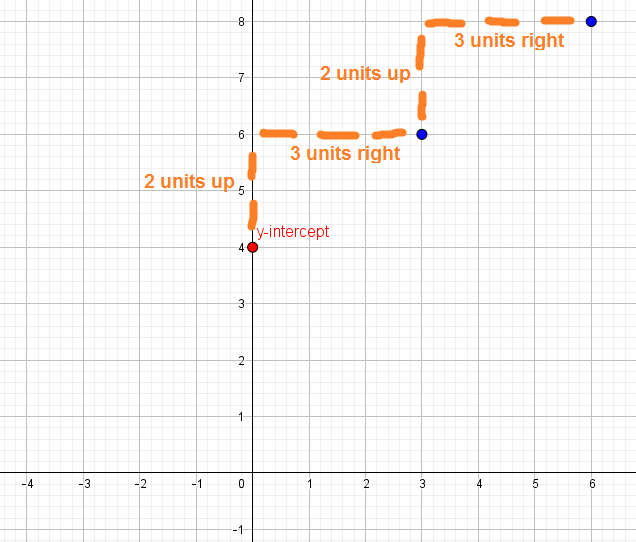Connect the points, and you have the graph of your linear function!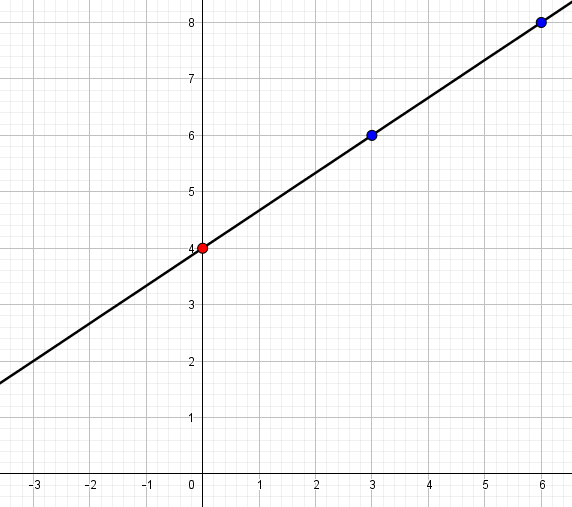This method for graphing linear equations can be applied even when the slope is negative or when the slope is not a fraction, even if it doesn’t seem that way. The next example will show you how that works!

### Example

Graph the linear equation:
$$y = -2x + 1$$

### Solution

The y-intercept here is 1, so plot this point first.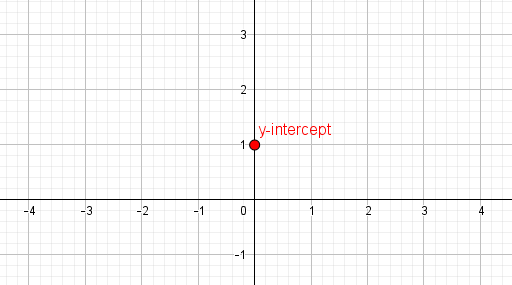The slope is –2. While this isn’t a fraction, it can be viewed as one if you let the denominator equal 1.

$$\text{slope} = -2 = \dfrac{-2}{1}$$

In other words:

$$\dfrac{-2}{1} = \dfrac{\text{down two units}}{\text{for every 1 unit right}}$$

Now this can be applied to find points on the graph.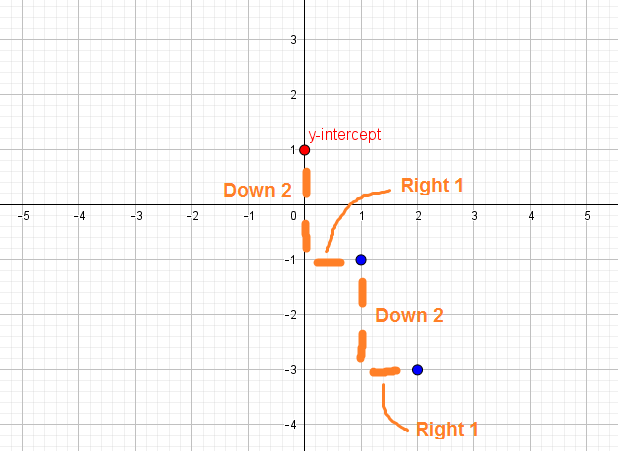Finally, connect the points to sketch the graph of the linear equation.TIP: If the slope is in decimal form, see if you can convert it to a fraction to apply this method. Otherwise, method 1 might be best.

## Method 3: Using the x- and y-intercepts

When graphing linear equations that are given in the form $$y = mx + b$$, it is easiest to just apply method 2. But sometimes, linear equations are given in standard form: $$Ax + By = C$$, where $$A$$, $$B$$, and $$C$$ are positive or negative whole numbers. In this case, using the x- and y-intercept may be the quickest approach.

### Example

Graph the linear equation:
$$-3x + 2y = 6$$

### Solution

To find the x-intercept, which is the point where the graph crosses the x-axis, let $$y = 0$$ and solve for $$x$$:

\begin{align}-3x + 2y &= 6\\ -3x + 2(0) &= 6\\-3x &= 6\\x &= -2\end{align}

To find the y-intercept, which is the point where the graph crosses the y-axis, let $$x = 0$$ and solve for $$y$$:

\begin{align}-3(0) + 2y &= 6\\2y &= 6\\y &= 3\end{align}

This gives you two points on the line, which you can plot and then connect to graph the linear equation.To read more about x-intercepts and y-intercepts, check out the articles Understanding x-intercepts and Understanding y-intercepts.

## Summary

When graphing linear equations, there are many possible approaches. Three common approaches are:

• Finding points:
pick simple values of $$x$$ and find the corresponding values of $$y$$. Plot these points and use these to graph your line.
• Using the slope and y-intercept:
use the concept of “rise over run” and the y-intercept to find points on the graph. This method is especially useful is the line is in slope-intercept form.
• Using the x- and y-intercepts:
let $$x = 0$$ and $$y = 0$$ to find the intercepts of the graph. Then, use these points to plot the line. This method is useful when the linear equation is in standard form.

Which method you use depends on the form of the linear equation you have and which method you are most comfortable with. No matter what, you can always find points if you get stuck.## Subscribe to our Newsletter!

We are always posting new free lessons and adding more study guides, calculator guides, and problem packs.

Sign up to get occasional emails (once every couple or three weeks) letting you know what's new!